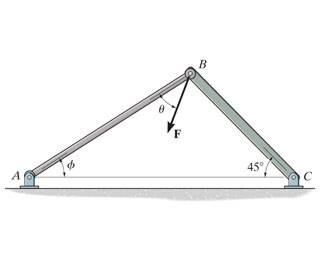# Finding an unknown force at an unknown angle

• Xovvo

## Homework Statement

So we have a force of unknown magnitude acting on these struts at an angle θ measured from strut AB.
The component of the force acting along AB is 600lb, and the magnitude of the force acting along BC is 500lb.
If Φ = 60°, what is the magnitude of F and the angle θ?Fcos(θ) = 600lb

## The Attempt at a Solution

Ok. So, it'll probably help if I knew the third angle of the triangle formed.
180° = ɣ + (60° + 45°)
180° - 105° = ɣ
75° = ɣ

Great. So, I know that Fcos(θ) = 600lb, and Fcos(75° - θ) = 500lb
hm. Fcos(θ)/600 = 1 = Fcos(75 - θ)/500
500Fcos(θ) = 600Fcos(75° - θ)
5Fcos(θ) = 6Fcos(75° - θ)
5cos(θ) = 6cos(75° - θ)
0 = 5cos(θ) - 6cos(75° - θ)

Originally I tried finding where z = 5cos(θ) - 6cos(75° - θ) intersected with z = θ + η where η = 75° + θ, but I couldn't get Wolfram Alpha to understand what I was talking about. Here, I see I should have just left η as
75° - θ, but even still, I have to *ask* Wolfram Alpha what θ works for 0 = 5cos(θ) - 6cos(75° - θ) when
0<=θ<=75° (it gives me an angle of ~30.7°).

Worse, since I couldn't figure it out, I figures if I gave in on the magnitude of F, I could still find the angle. Mastering Engineering told me F = 870lb. So, if Fcos(θ) = 600, θ=arccos(600/F) and arccos(600/870) ≈ 46.4°.
Which was wrong. The θ it wanted was ~34°

Which means everything I did was wrong.
So what triangle magic do I do to get from the initial problem, to the final F=870 θ=34°, without doing something so complicated I need Wolfram Alpha to crunch it out?

A hint: cos(x-y) = cos(x)cos(y) + sin(x)sin(y). Apply that to cos(75° - θ).

Great. So, I know that Fcos(θ) = 600lb, and Fcos(75° - θ) = 500lb
Good. I suggest using a trig identity to simplify the expression Fcos(75° - θ).

While that does get everything in simpler terms of θ, that doesn't address that 870cos(34) = 721.3 and not 600.
My error is a lot farther up.

While that does get everything in simpler terms of θ, that doesn't address that 870cos(34) = 721.3 and not 600.
My error is a lot farther up.
Start over with the two simpler equations. You'll get a different value for θ and F.

what do you mean by that? Because F=870 and θ≈34° are set in stone Those are the answers for this problem.

what do you mean by that? Because F=870 and θ≈34° are set in stone
And yet you know that:
870cos(34) = 721.3 and not 600.

Those are the answers for this problem.
Says who?

Why not just solve it using that trig substitution. You'll solve it easily without needing Wolfram.

Says who?
Says The homework. Because I've already gotten this question wrong, and the answers were displayed. I'm asking about this question here so I know what to do when this sort of problem comes up again.

Says The homework. Because I've already gotten this question wrong, and the answers were displayed.
Do you not agree that the given answers do not work? That they contradict the problem statement?